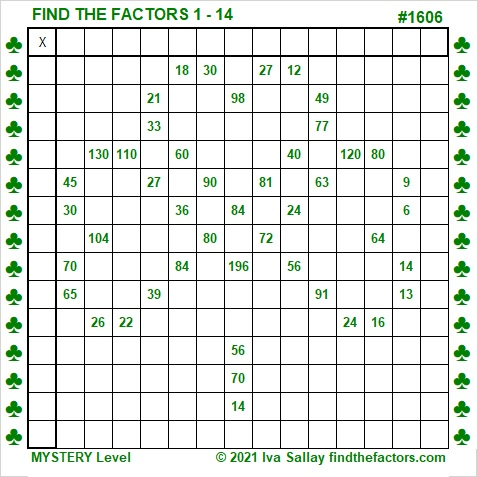# 1606 A Lucky Shamrock

Contents

### Today’s Puzzle:

Even if you don’t know all the factors of some of these clues in this shamrock puzzle, there are enough that you will know, and you can figure the rest out easily. Lucky you!Print the puzzles or type the solution in this excel file: 14 Factors 1604-1612.

### Factors of 1606:

• 1606 is a composite number.
• Prime factorization: 1606 = 2 × 11 × 73.
• 1606 has no exponents greater than 1 in its prime factorization, so √1606 cannot be simplified.
• The exponents in the prime factorization are 1, 1, and 1. Adding one to each exponent and multiplying we get (1 + 1)(1 + 1)(1 + 1) = 2 × 2 × 2 = 8. Therefore 1606 has exactly 8 factors.
• The factors of 1606 are outlined with their factor pair partners in the graphic below.### More about the Number 1606:

1606 is the hypotenuse of a Pythagorean triple:
1056-1210-1606. which is 22 times (48-55-73).

1606 is in a couple of other Pythagorean triples that can be calculated from
2(803)(1), 803² – 1², 803² + 1², and
2(73)(11), 73² – 11², 73² + 11².

This site uses Akismet to reduce spam. Learn how your comment data is processed.